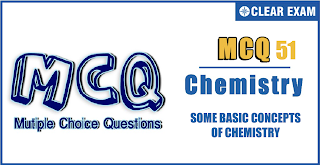## SOME BASIC CONCEPTS OF CHEMISTRY Quiz-51

IIT JEE exam which consists of JEE Main and JEE Advanced is one of the most important entrance exams for engineering aspirants. The exam is held for candidates who are aspiring to pursue a career in the field of engineering and technical studies. Chemistry is important because everything you do is chemistry! Even your body is made of chemicals. Chemical reactions occur when you breathe, eat, or just sit there reading. All matter is made of chemicals, so the importance of chemistry is that it's the study of everything..

Q1.The calcium in a 0.8432 g sample is precipitated as CaC_2 O_4. The precipitate is washed, ignited to CaCO_3 and found to weigh 0.3462 g. percentage of CaO in the sample is
•  11.5%
•  23%
•  18%
•  46%
Q2.CaCO_3 is decomposed by HCl (density 1.825 g/cc) CaCO_3+2HCl→CaCl_2+H_2 O+CO_2 Volume of HCl required to decompose 10 g of 50% pure CaCO_3 is a)
•  1.825 mL
•  3.65 mL
•  0.9125 mL
•  2 mL
Solution

(d) CaCO_3+2HCl→CaCl_2+H_2 O+CO_2 100 g 73 g 10 g 7.3 g (50% pure) 5 g 7.3/2 g HCl mass/volume=density ∴Volume of HCl=(mass of HCl)/(density of HCl)=7.3/(2×1.825)=2 mL

Q3.  A mixture contains 1 mol each of Fe^(2+) and Fe^(3+)ions. Volume of 1 M MnO_4^- required by the mixture in the oxidation of the given mixture is
•  400 mL
•  200 mL
•  100 mL
•  1000 mL
•  A
•  B
•  C
•  D
Q5.0.116 g of C_4 H_4 O_4 (A) is neutralized by 0.074 g of Ca(OH)_2.Hence, protonic hydrogen (H^+ ) in (A)will be
•  1
•  2
•  3
•  4
Solution
(b) 0.116 g C_4 H_4 O_4 (A)=0.116/116=0.001 mol =0.001 x equivalent 0.074 g Ca(OH)_2=0.074/74=0.001mol =0.002 equivalent ∴0.001x=0.002 ∴x=2

Q6. SO_2 and CO_2 both turn lime water (A) milky, SO_2 also turns K_2 Cr_2 O_7/H^+ (B) green while O_2 is soluble in pyrogallol (C) turning it black. These gases are to be detected in order by using these reagents in a mixture. The order is
•  (a),(b),(c)
•  (b),(c),(a)
•  (b),(a),(c)
•  (a),(c),(b)
Q7.Mixture X = 0.02 mole of [Co(NH_3 )_5 SO_4]Br and 0.02 mole of [Co(NH_3 )_5 Br] SO_4 was prepared in 2 L of solution. 1 L of mixture X+excess AgNO_3→Y 1 L of mixture X+excess BaCl_2→Z Number of moles of Y and Z are
•  0.01, 0.01
•  0.02, 0.01
•  0.01, 0.02
•  0.02, 0.02
•  A
•  B
•  C
•  D
Q9.A sample of CuSO_4∙5H_2 O contains 3.782 g of Cu. How many grams of oxygen are in this sample? (Cu = 63.5)
•  0.952 g
•  3.80 g
•  4.761 g
•  8.576 g
Solution
(d) x mol CuSO_4.5H_2 O =249.5x g CuSO_4.5H_2 O =63.5 x g Cu =3.782 g Cu ∴63.5 x=3.782 x=0.05956 mol Every 1 mole of salt has =9 moles O-atoms ∴O-atoms=0.05956 x 9 mol =0.05956×9×16 g =8.576 g O-atoms

Q10.10 mL of H_2 O_2 solution (volume strength =x) required 10 mL of N/0.56 Mn〖O_4〗^⊖ solution in acidic medium. Hence, x is:
•  0.56
•  5.6
•  0.1
•  10
Solution
(d) N_1 (H_2 O_2 )=1/0.56 ‘x volume’ H_2 O_2 has normality =x/5.6 x/5.6=1/0.56,⇒ x=10#### Written by: AUTHORNAME

AUTHORDESCRIPTION## Want to know more

Please fill in the details below:

## Latest NEET Articles\$type=three\$c=3\$author=hide\$comment=hide\$rm=hide\$date=hide\$snippet=hide

Name

ltr
item
BEST NEET COACHING CENTER | BEST IIT JEE COACHING INSTITUTE | BEST NEET & IIT JEE COACHING: SOME BASIC CONCEPTS OF CHEMISTRY Quiz-51
SOME BASIC CONCEPTS OF CHEMISTRY Quiz-51
https://1.bp.blogspot.com/-XvTVLEfBjvo/YOSzL7vk03I/AAAAAAAAUCo/VanB8mZCLVYA4q04s7PAOxrluhKqcGSuACLcBGAsYHQ/s320/Screenshot%2B2021-07-06%2Bat%2B6.34.56%2BPM.png
https://1.bp.blogspot.com/-XvTVLEfBjvo/YOSzL7vk03I/AAAAAAAAUCo/VanB8mZCLVYA4q04s7PAOxrluhKqcGSuACLcBGAsYHQ/s72-c/Screenshot%2B2021-07-06%2Bat%2B6.34.56%2BPM.png
BEST NEET COACHING CENTER | BEST IIT JEE COACHING INSTITUTE | BEST NEET & IIT JEE COACHING
https://www.cleariitmedical.com/2021/07/some-basic-concepts-of-chemistry-quiz-51.html
https://www.cleariitmedical.com/
https://www.cleariitmedical.com/
https://www.cleariitmedical.com/2021/07/some-basic-concepts-of-chemistry-quiz-51.html
true
7783647550433378923
UTF-8

STAY CONNECTED